﻿ 减污降碳协同推进与中国3E绩效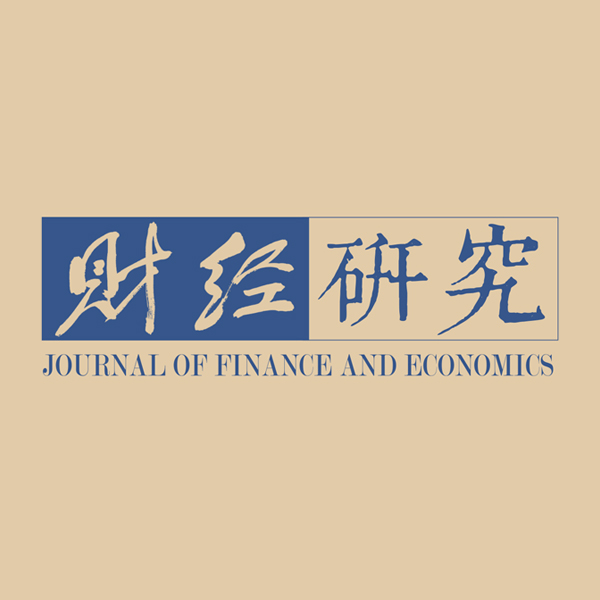《财经研究》
2022第48卷第9期

1. 山东财经大学 经济学院，山东 济南 250014;
2. 厦门大学 王亚南经济研究院，福建 厦门 361005;
3. 东南大学 经济管理学院，江苏 南京 211189

Coordinated Promotion of Pollution and Carbon Reduction and China’s 3E Performance
Liu Huajun1, Qiao Liecheng2, Guo Lixiang3
1. School of Economics, Shandong University of Finance and Economics, Jinan 250014, China;
2. The Wan Yanan Institute for Studies in Economics, Xiamen University, Xiamen 361005, China;
3. School of Economics and Management, Southeast University, Nanjing 211189, China
Summary: The construction of ecological civilization is a fundamental plan related to the sustainable development of China. During the “14th Five-Year Plan” period, China’s ecological civilization construction has entered a key period focusing on carbon reduction, promoting the co-benefits of pollution and carbon reduction, promoting the comprehensive green transformation of economic and social development, and realizing the improvement of ecological environment quality from quantitative change to qualitative change. Meanwhile, it is also facing the severe challenges brought by carbon peak and carbon neutralization, as well as the arduous task of thoroughly fighting the tough battle of pollution prevention and control. Standing at a new historical point, we must more completely, accurately and comprehensively implement the concept of Two Mountains Theory, comprehensively promote pollution control, ecological protection and climate change, promote the co-benefits of pollution and carbon reduction, accelerate economic and social development, and realize comprehensive green transformation. This paper brings air pollution and carbon emissions into the 3E (energy-environment-economy) performance research framework. Through the multi-dimensional comparative analysis of time, space and subsystems, this paper quantitatively investigates the impact of coordinated promotion of pollution and carbon reduction on the performance of China’s 3E system and its subsystems. The results show that: During the sample investigation period, the coordinated promotion of pollution and carbon reduction has increased the overall performance of China’s 3E system by 0.91% per year. Especially since the 18th CPC National Congress, the coordinated promotion of pollution and carbon reduction has played a more significant role in improving the performance. At the level of 3E subsystem, the coordinated promotion of pollution and carbon reduction is conducive to the performance improvement of economic system, energy system and environmental system, but the performance improvement of energy system faces greater challenges. The marginal contribution of this paper may be reflected in the following aspects: First, this paper brings the coordinated promotion of pollution and carbon reduction into the 3E performance research framework for the first time, which enriches the theoretical research system of coordinated control of pollution and carbon reduction. Second, this paper gives full play to the additive characteristics of Luenberger productivity index and puts forward the calculation idea of energy, environment and economic subsystem performance. Third, this paper shows the great achievements of China’s ecological civilization construction since the 18th CPC National Congress, and deepens the understanding of the idea that lucid waters and lush mountains are invaluable assets.
Key words: pollution and carbon reduction    3E system    performance evaluation    Two Mountains Theory

“绿水青山就是金山银山”，是新发展理念的重要内容，是习近平生态文明思想的核心内容，为新时代生态文明建设和美丽中国建设提供了基本遵循，拥有持久的生命力和强大的引领力。协同推进减污降碳，必须以“两山”理念为指引，深刻认识并准确把握“两山”理念的精神实质和科学内涵。首先，新发展阶段的发展是高质量发展。推动实现高质量发展，不是简单地以GDP论英雄，而是强调以提高经济质量和效益为立足点。在新发展阶段，既要绿水青山，又要金山银山，而且绿水青山就是金山银山。减污降碳协同推进在促进创新、节约资源等方面有诸多益处，要从系统、整体的角度看待减污降碳协同推进对于实现经济社会发展全面绿色转型的重大意义。其次，绿色发展是推动高质量发展的题中之义。绿色发展注重实现经济建设、资源供给、环境保护等多方面协调发展。就减污降碳对经济社会的影响而言，涉及多个系统、涵盖多个部门，不能只盯着经济发展这个小账，而是要兼顾能源绩效、环境绩效，算好生态文明建设全局的大账、整体账、长远账。最后，绿色低碳发展必须处理好短期和中长期的关系。实现碳达峰、碳中和是一场广泛而深刻的经济社会系统性变革，尽管短期内将不可避免地经历阵痛，但从长期来看这是经济社会发展绿色转型的必然过程。绿色低碳发展不仅是历史发展的大趋势，也是时代的要求、前进的方向。虽有智慧，不如乘势。面对新的历史任务，必须顺应历史潮流，积极应变、主动求变，以最小的成本实现成功的转型和未来更好的发展；若不能顺应时代的潮流，即使得到金山银山，也是不可持续的金山银山，坐等观望，等到的只有更高的转型成本和未来更难的发展。

3EEnergy-Environment-Economy）系统是能源、环境和经济子系统在互相作用、互相渗透、互相联系下形成的结构和功能相统一的动态复杂系统（兰天阳，2016）。从系统论角度出发，3E绩效是既能衡量3E系统整体发展水平的动态变动，又可以反映能源、环境和经济三者作为一个有机整体耦合协调程度的经济学概念。相关研究将环境约束引入3E系统，并通过比较引入环境约束后绩效水平的变化来判断环境污染治理对绩效的影响。目前存在以下三种观点：第一种观点认为环境污染治理会对经济绩效造成损失（Watanabe和Tanaka，2007；陈诗一，2010；Xia和Xu，2020），即生态环境保护占用了原本用于生产的资源，从而降低经济绩效，并且生产过程采用不环保的技术，由此产生的污染排放造成大量的外部成本。第二种观点则认为考虑环境因素会提高经济绩效（王兵等，2010；黄清煌和高明，2016；Meng和Qu，2022），并且运用“波特假说”来解释考虑环境约束后的绩效提升。第三种观点认为环境治理对绩效的影响存在时效性，短期为正向推动作用，长期则表现出显著的负向影响（黄庆华等，2018；金刚和沈坤荣，2018）。上述研究的时代背景不同，指标选取和模型选取上也各具特点，因此在研究结论上存在明显差异。

（一）模型设定

1. 绩效评价模型的选择。参考Fukuyama和Weber（2009）的研究，本文在CRS假设下构造考虑非期望产出的SBM-DDF模型。该模型是一种非径向、非角度的DEA模型，不仅能够使投入和产出按照不同的比例进行缩减或扩张，还可以将资源环境约束纳入到效率测度模型中，提高了测度的准确性，使得效率测度模型涵盖了非期望产出因素。t时期决策单元DMUo在全局基准技术下的SBM-DDF模型如公式（1）所示：

 $\begin{array}{l}\stackrel{\rightharpoonup }{S}({x}_{o}^{t},{y}_{o}^{t},{b}_{o}^{t};{g}^{x},{g}^{y},{g}^{b})=\underset{{s}^{x},{s}^{y},{s}^{b},\lambda }{\mathrm{max}}\;\dfrac{1}{2}\left[\dfrac{1}{N}\sum _{n=1}^{N}\frac{{s}_{no}^{x}}{{g}_{n}^{x}}}+\dfrac{1}{M+Q}\left(\sum _{m=1}^{M}\frac{{s}_{mo}^{y}}{{g}_{m}^{y}}+\sum _{q=1}^{Q}\frac{{s}_{qo}^{b}}{{g}_{q}^{b}}}}\right)\right]\\ \begin{array}{ccccc}& & & & \end{array}\begin{array}{c}\end{array}s.t.\begin{array}{ccc}& & {x}_{no}^{t}\text=\sum _{t=1}^{T}\sum _{k=1}^{K}{\lambda }_{k}^{t}{x}_{nk}^{t}+{s}_{no}^{x},\forall n;}}\end{array}\\ \begin{array}{ccccc}& & & & \end{array}\begin{array}{c}\begin{array}{c}\end{array}\end{array}\begin{array}{c}\end{array}\begin{array}{c}\end{array}\begin{array}{ccc}& & {y}_{mo}^{t}\text=\sum _{t=1}^{T}\sum _{k=1}^{K}{\lambda }_{k}^{t}{y}_{mk}^{t}-{s}_{mo}^{y},\forall m;}}\end{array}\\ \begin{array}{ccccc}& & & & \end{array}\begin{array}{c}\begin{array}{c}\end{array}\end{array}\begin{array}{c}\end{array}\begin{array}{c}\end{array}\begin{array}{ccc}& & {b}_{qo}^{t}\text=\sum _{t=1}^{T}\sum _{k=1}^{K}{\lambda }_{k}^{t}{b}_{qk}^{t}+{s}_{qo}^{b},\forall q;}}\end{array}\\ \begin{array}{ccccc}& & & & \end{array}\begin{array}{c}\begin{array}{c}\end{array}\end{array}\begin{array}{c}\end{array}\begin{array}{c}\end{array}\begin{array}{ccc}& & {s}_{no}^{x}\end{array}\ge 0,{s}_{mo}^{y}\ge 0,{s}_{qo}^{b}\ge 0,{\lambda }_{k}^{t}\ge 0.\end{array}$ (1)

 $IE = IE_{Energy}^G + IE_{Labour}^G + IE_{Capital}^G + IE_{GDP}^G + IE_{Carbon}^G + IE_{Pollution}^G$ (2)

2. 卢恩伯格生产率指数的构建与分解。卢恩伯格生产率指数具有差分形式的相加结构，能够对整体绩效进行分解，可以刻画能源、环境和经济子系统绩效之间内在的逻辑关系。参考Chamber等（1996）和Oh（2010）的全局基准技术思路，本文构建了全局参比的卢恩伯格生产率指数（L），计算过程如公式（3）所示：

 $L_t^{t + 1} = IE_{}^G\left( {{x^t},{y^t},{b^t};{g_x},{g_y},{g_b}} \right) - IE_{}^G\left( {{x^{t + 1}},{y^{t + 1}},{b^{t + 1}};{g_x},{g_y},{g_b}} \right)$ (3)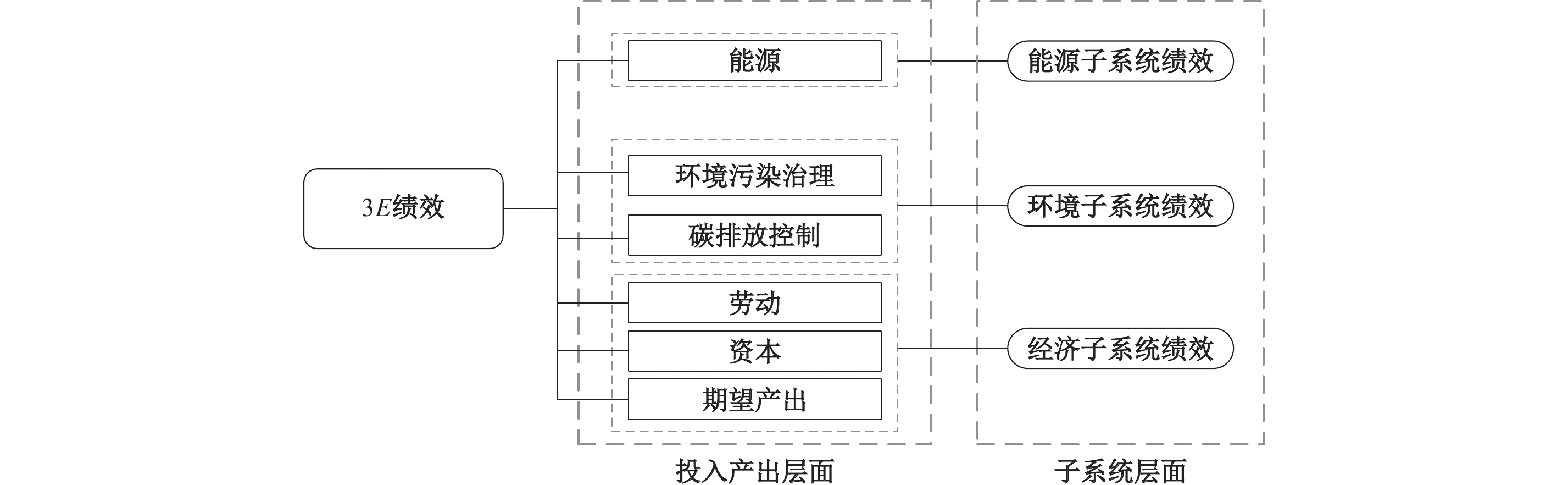图 1 3E绩效分解思路

 $L_t^{t + 1} = {\left( {L_t^{t + 1}} \right)_{Energy}} + {\left( {L_t^{t + 1}} \right)_{Labour}} + {\left( {L_t^{t + 1}} \right)_{Capital}} + {\left( {L_t^{t + 1}} \right)_{GDP}} + {\left( {L_t^{t + 1}} \right)_{Pollution}} + {\left( {L_t^{t + 1}} \right)_{Carbon}}$ (4)

 $L_t^{t + 1} = {\left( {L_t^{t + 1}} \right)_{Energy}} + {\left( {L_t^{t + 1}} \right)_{Environment}} + {\left( {L_t^{t + 1}} \right)_{Economy}}$ (5)
 ${\left( {L_t^{t + 1}} \right)_{Energy}} = IE_{Energy}^G\left( {{x^t},{y^t},{b^t};{g_x},{g_y},{g_b}} \right) - IE_{Energy}^G\left( {{x^{t + 1}},{y^{t + 1}},{b^{t + 1}};{g_x},{g_y},{g_b}} \right)$ (6)
 $\begin{gathered} {\left( {L_t^{t + 1}} \right)_{Environment}} = {\left( {L_t^{t + 1}} \right)_{Pollution}} + {\left( {L_t^{t + 1}} \right)_{Carbon}} \\ = IE_{Pollution}^G\left( {{x^t},{y^t},{b^t};{g_x},{g_y},{g_b}} \right) - IE_{Pollution}^G\left( {{x^{t + 1}},{y^{t + 1}},{b^{t + 1}};{g_x},{g_y},{g_b}} \right) \\ + IE_{Carbon}^G\left( {{x^t},{y^t},{b^t};{g_x},{g_y},{g_b}} \right) - IE_{Carbon}^G\left( {{x^{t + 1}},{y^{t + 1}},{b^{t + 1}};{g_x},{g_y},{g_b}} \right) \\ \end{gathered}$ (7)
 $\begin{gathered} {\left( {L_t^{t + 1}} \right)_{Economy}} = {\left( {L_t^{t + 1}} \right)_{Capital}} + {\left( {L_t^{t + 1}} \right)_{Labour}} + {\left( {L_t^{t + 1}} \right)_{GDP}} \\ = IE_{Capital}^G\left( {{x^t},{y^t},{b^t};{g_x},{g_y},{g_b}} \right) - IE_{Capital}^G\left( {{x^{t + 1}},{y^{t + 1}},{b^{t + 1}};{g_x},{g_y},{g_b}} \right) \\ + IE_{Labour}^G\left( {{x^t},{y^t},{b^t};{g_x},{g_y},{g_b}} \right) - IE_{Labour}^G\left( {{x^{t + 1}},{y^{t + 1}},{b^{t + 1}};{g_x},{g_y},{g_b}} \right) \\ + IE_{GDP}^G\left( {{x^t},{y^t},{b^t};{g_x},{g_y},{g_b}} \right) - IE_{GDP}^G\left( {{x^{t + 1}},{y^{t + 1}},{b^{t + 1}};{g_x},{g_y},{g_b}} \right) \\ \end{gathered}$ (8)

（二）数据说明

（一）减污降碳协同推进与中国3E绩效：全时期考察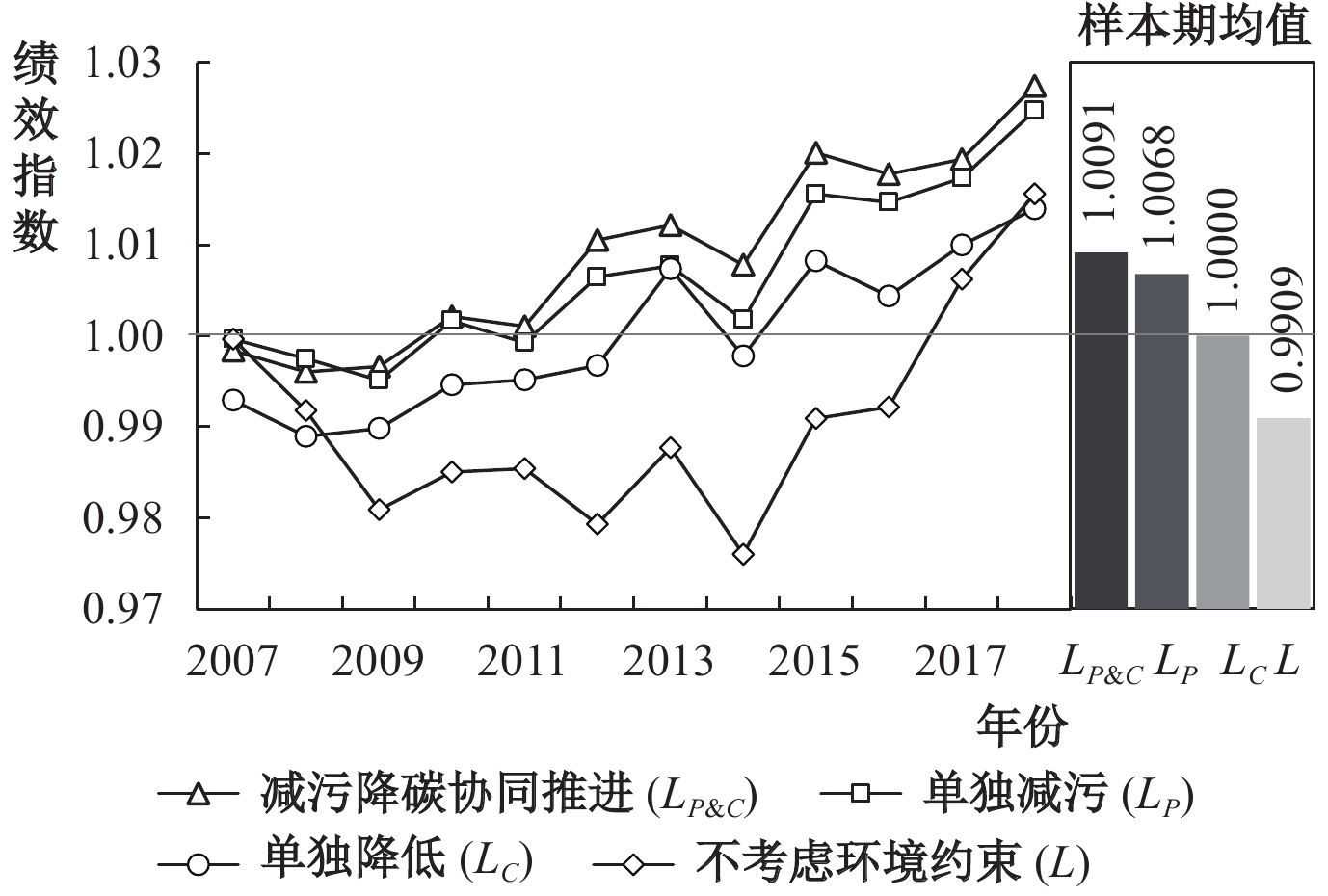图 2 中国3E绩效的时序变化与样本期均值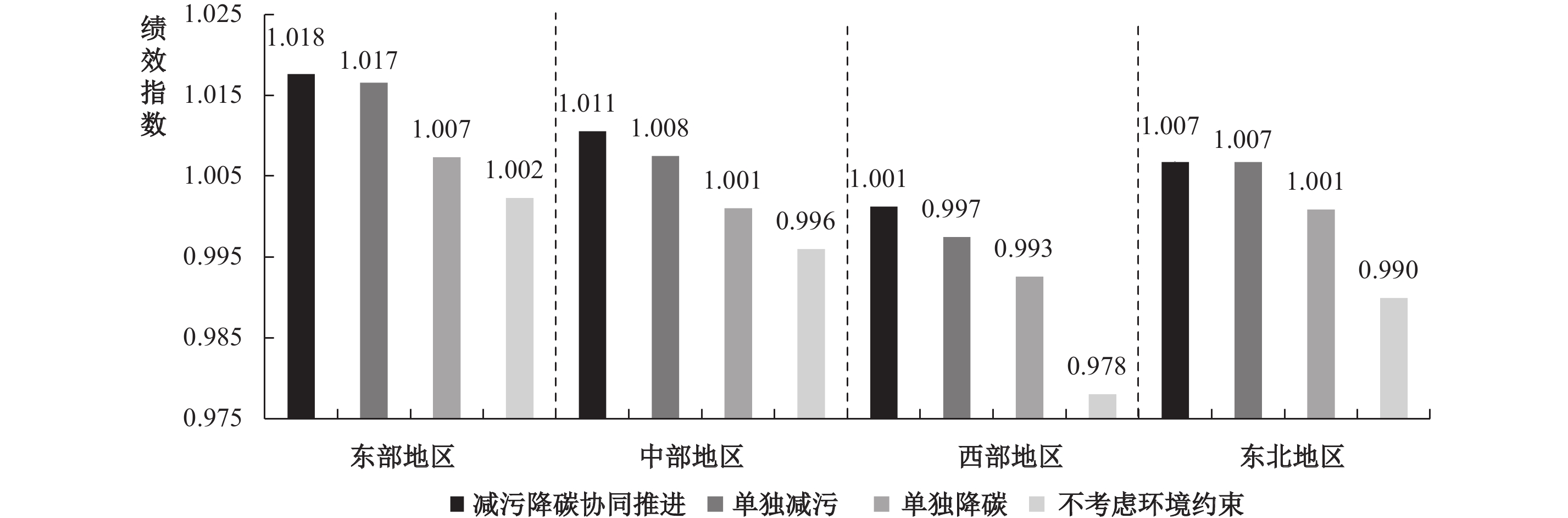图 3 中国四大区域3E绩效

（二）减污降碳协同推进与中国3E绩效：阶段性考察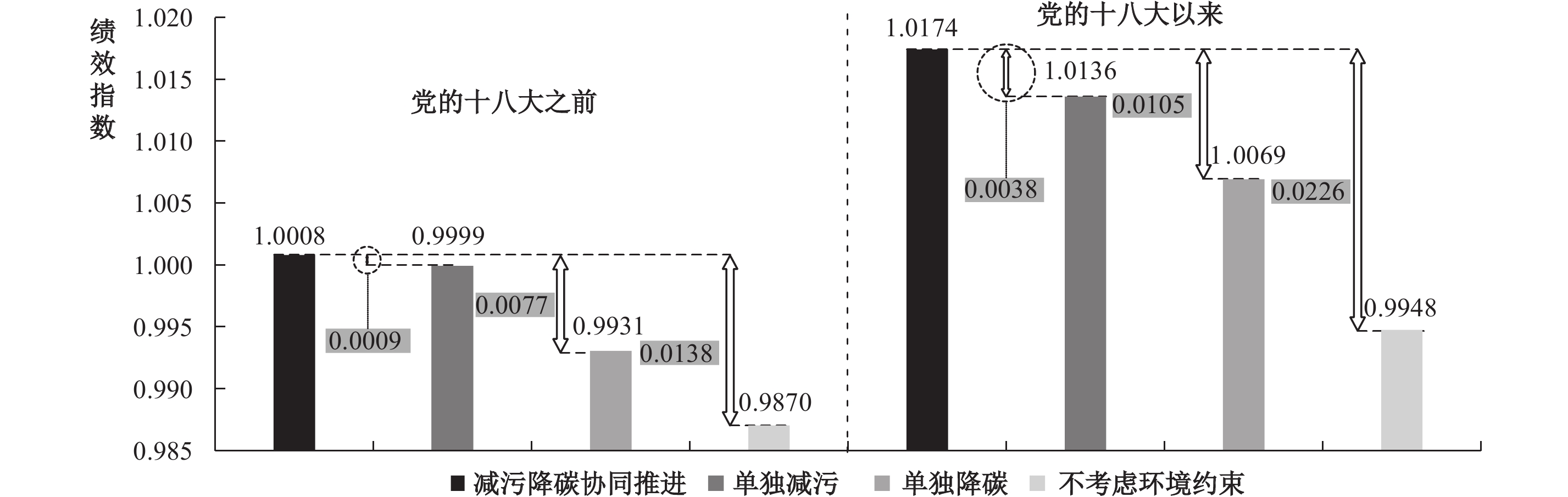图 4 党的十八大前后中国3E绩效对比

 区域 减污降碳 单独减污 单独降碳 不考虑环境约束 环保提升绩效 前 后 前 后 前 后 前 后 前 后 变化 东部 1.0115 1.0238 1.0107 1.0225 1.0033 1.0113 0.9998 1.0048 0.0117 0.0190 +0.0073 中部 1.0012 1.0199 1.0011 1.0139 0.9938 1.0082 0.9920 1.0000 0.0092 0.0199 +0.0107 西部 0.9908 1.0117 0.9903 1.0047 0.9842 1.0010 0.9773 0.9788 0.0135 0.0329 +0.0194 东北 1.0009 1.0125 0.9973 1.0162 0.9900 1.0118 0.9706 1.0093 0.0303 0.0032 −0.0271 注：“前”“后”分别代表党的十八大之前和党的十八大以来的3E绩效;“环保提升绩效”表示减污降碳协同推进与不考虑环境约束时的绩效差值。

（一）全国层面3E子系统绩效的整体水平及阶段性特征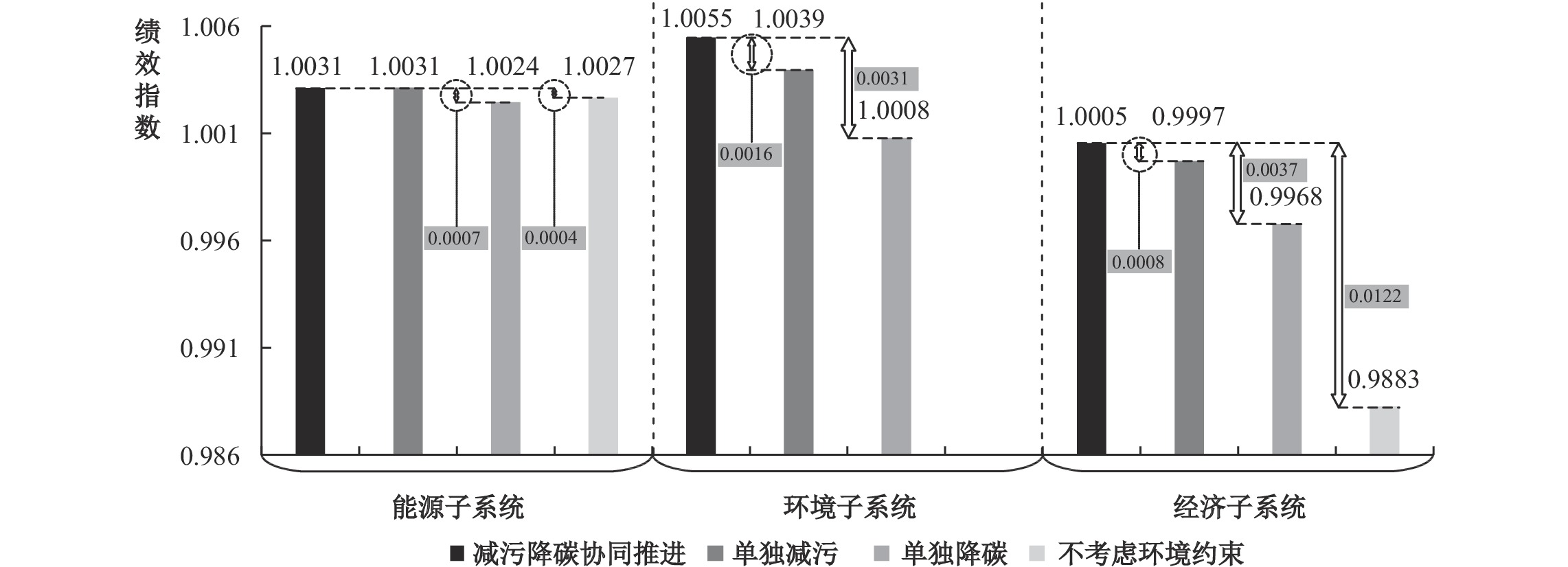图 5 2006—2018年中国能源、环境和经济子系统绩效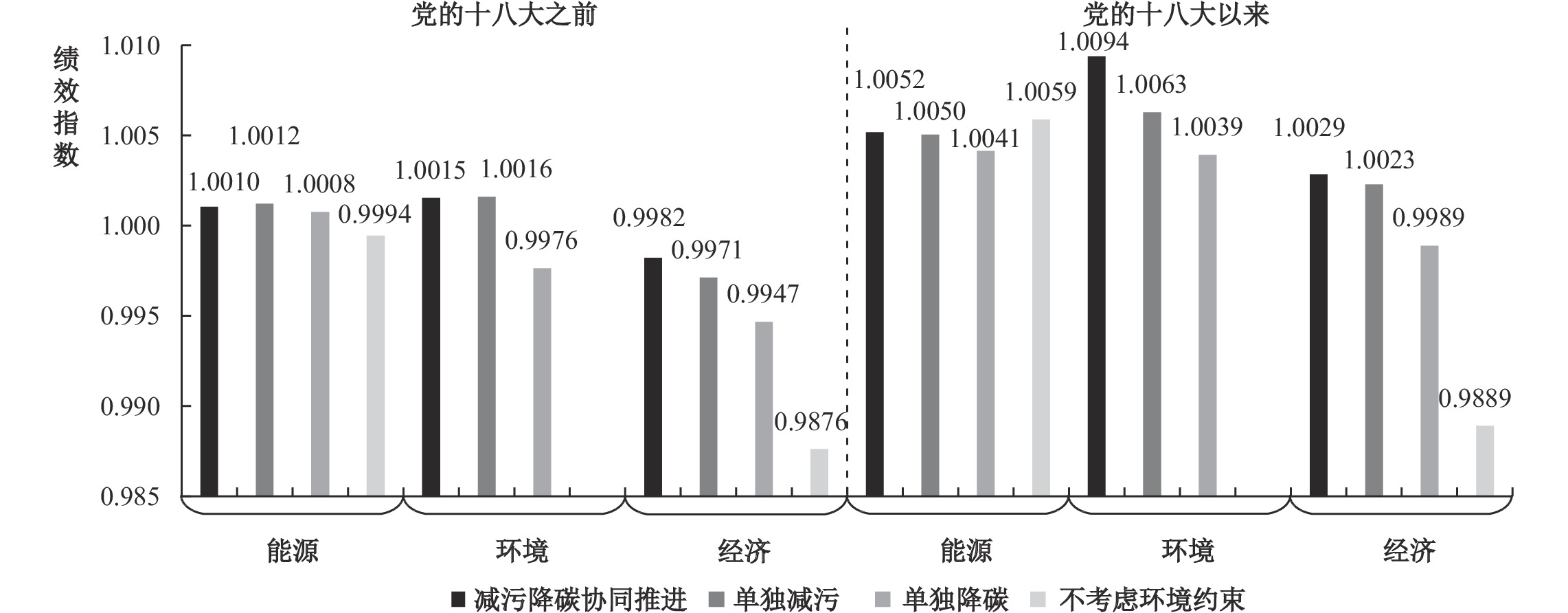图 6 党的十八大前后中国能源、环境和经济子系统绩效对比

（二） 区域层面3E子系统绩效的整体水平及阶段性特征

 子系统 区域 全时期 十八大前 十八大后 污碳 污 碳 无 污碳 污 碳 无 污碳 污 碳 无 能源 东部 1.0043 1.0040 1.0030 1.0041 1.0029 1.0029 1.0016 1.0023 1.0056 1.0050 1.0044 1.0059 中部 1.0039 1.0043 1.0024 1.0025 1.0008 1.0019 1.0004 0.9961 1.0070 1.0068 1.0043 1.0088 西部 1.0017 1.0021 1.0018 1.0017 0.9996 0.9989 1.0001 0.9998 1.0039 1.0053 1.0035 1.0037 东北 1.0028 1.0018 1.0029 1.0016 1.0008 1.0028 1.0008 0.9953 1.0048 1.0008 1.0050 1.0079 环境 东部 1.0092 1.0085 1.0024 — 1.0048 1.0052 0.9994 — 1.0136 1.0117 1.0054 — 中部 1.0060 1.0022 1.0003 — 1.0039 1.0008 0.9976 — 1.0081 1.0036 1.0031 — 西部 1.0025 1.0018 0.9996 — 0.9976 0.9992 0.9959 — 1.0073 1.0043 1.0034 — 东北 1.0031 1.0005 1.0006 — 1.0005 0.9997 0.9985 — 1.0056 1.0013 1.0026 — 经济 东部 1.0042 1.0041 1.0019 0.9982 1.0039 1.0025 1.0023 0.9975 1.0046 1.0057 1.0014 0.9989 中部 1.0007 1.0010 0.9983 0.9935 0.9966 0.9984 0.9958 0.9958 1.0048 1.0036 1.0008 0.9912 西部 0.9970 0.9937 0.9911 0.9763 0.9936 0.9922 0.9882 0.9775 1.0004 0.9952 0.9940 0.9751 东北 1.0009 1.0045 0.9974 0.9883 0.9996 0.9949 0.9906 0.9753 1.0021 1.0141 1.0042 1.0014 注：“污碳”“污”“碳”“无”分别表示减污降碳协同推进、单独减污、单独降碳、不考虑环境约束的绩效指数。

① 将样本考察期设定为2006—2018年，主要基于以下考虑：一是在《国民经济和社会发展第十一个五年规划纲要》中，党和国家历史上第一次将“环境友好型社会”载入经济社会发展文件，这是生态文明建设执政理念的一大突破，为此，本文选择以2006年为研究起点；二是将2018年设定为样本期终点，既保证了所有投入产出数据均可完整获取，又最大可能地兼顾了本研究的时效性、前沿性。

② 圣路易斯华盛顿大学大气成分分析小组网址：https://sites.wustl.edu/acag/datasets/surface-pm2-5。